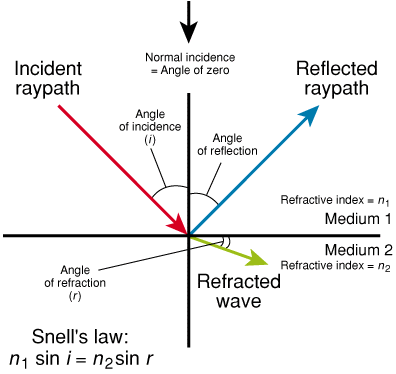# Explore the Energy Glossary

Look up terms beginning with:

# Snell's law

1. n. [Geophysics]

The mathematical description of refraction, or the physical change in the direction of a wavefront as it travels from one medium to another with a change in velocity and partial conversion and reflection of a P-wave to an S-wave at the interface of the two media. Snell's law, one of two laws describing refraction, was formulated in the context of light waves, but is applicable to seismic waves. It is named for Willebrord Snel (1580 to 1626), a Dutch mathematician.

Snell's law can be written as:

n1 sin i = n2 sin r,

where
n1 = refractive index of first medium
n2 = refractive index of second medium
sin i = sine of the angle of incidence
sin r = sine of the angle of refraction.Diagram of reflection and refraction.